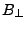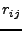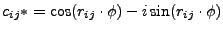Next: SLANT2H Up: Implementation Previous: Implementation   Contents

## Algorithm

Input is the unwrapped topo-interferogram (corrected for 'flat earth'). Format is hgt, or real4. Not unwrapped thus indicated by NaN==-999. (real4) or if amplitude==0 (hgt). Defo-interferogram is wrapped (complex real4, mph) (specified in interferogram result file).

1. Obtain orbit for topo-slave (result file). Obtain filename/dimension of unwrapped interferogram (result file).
2. Read in matrices, appropriate size/format etc. per line. Check if they exactly overlap.
3. Computeandon a small grid (20x10 points over the image).
4. Model the ratio of the perpendicular baselines by a 2D polynomial of degree 1. (r(l,p) = a00 + a10l + a01p) Give statistics for max. error due to modelling. (It seems the ratio hardly changes over the image for ERS1/2).
5. Compute wrapped deformation phase (phase corrected for topography) with formula 31.17, using the modeled ratio. (Actually compute it complex:.)
6. Set not unwrapped regions to (0.,0.)
7. Write output file (complex real4, mph format). (if a problem with unwrapping occurred, write (0,0).) If requested, also write the scaled unwrapped interferogram.

Leijen 2009-04-14